Everyday maths 2 (Wales)

Start this free course now. Just create an account and sign in. Enrol and complete the course for a free statement of participation or digital badge if available.

Free course

# 10.5 Other applications of ratio

A very common and practical use of ratio is when you want to change the proportions of a recipe. All recipes state the number of portions they will make, but this is not always the number that you wish to make.You may wish to make more or less than the actual recipe gives. If you wanted to make 18 scones but only have a recipe that makes 12, how do you know how much of each ingredient to use?

• To make 12 scones
• 400 g self-raising flour
• 1 tablespoon caster sugar
• 80 g butter
• 250 ml milk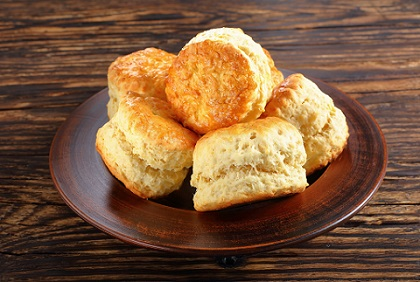Figure 19 Scones on a plate

As you already know the ingredients to make 12 scones, you need to know how much of each ingredient to make an extra 6 scones. Since 6 is half of 12, if you halve each ingredient, you will have the ingredients for the extra 6 scones. To find the total for 18 scones you need to add together the ingredients for the 12 scones and the 6 scones.

## Table 9

 12 scones 6 scones 18 scones 400 g flour 400 g ÷ 2 = 200 g flour 400 g + 200 g = 600 g flour 1 tablespoon caster sugar 1 ÷ 2 = one divided by two tablespoon caster sugar 1 + one divided by two = 1 one divided by two tablespoons caster sugar 80 g butter 80 g ÷ 2 = 40 g butter 80 g + 40 g = 120 g butter 250 ml milk 250 ml ÷ 2 = 125 ml milk 250 ml + 125 ml = 375 ml milk

Have a go at the activity below to check your skills.

## Activity 28: Ratio and recipes

1. This recipe makes 18 biscuits:

• 220 g self-raising flour
• 150 g butter
• 100 g caster sugar
• 2 eggs
• How much of each ingredient is needed for 9 biscuits?

1. Since 9 is half of 18, you need to halve each ingredient to find the amount required to make 9 biscuits.

• 220g ÷ 2 = 110 g flour
• 150g ÷ 2 = 75 g butter
• 100g ÷ 2 = 50 g sugar
• 2 ÷ 2 = 1 egg
1. To make strawberry milkshake you need:
• 630 ml milk
• 3 scoops of ice cream
• 240 g of strawberries
• The recipe serves 3
• How much of each ingredient is needed for 9 people?

1. You know the ingredients for 3 but want to know the ingredients for 9. Since 9 is three times as big as 3, you need to multiply each ingredient by 3.
• 630 ml × 3 = 1890 ml milk
• 3 × 3 = 9 scoops of ice cream
• 240 g × 3 = 720 g of strawberries
1. Angel Delight recipe:
• Add 60 g powder to 300 ml cold milk
• Serves 2 people
• How much of each ingredient is needed to serve 5 people?

1. You could work this out in 2 different ways.

Method 1

You know the ingredients for 2 people. You can find ingredients for 4 people by doubling the ingredients for 2. You then need ingredients for an extra 1 person. Since 1 is half of 2, you can halve the ingredients for 2 people.

• 60 g + 60 g + 30 g = 150 g powder
• 300 ml + 300 ml + 150 ml = 750 ml milk

Method 2

You know the ingredients for 2 people so you can find the ingredients for 1 person by halving them. You can then multiply the ingredients for 1 person by 5.

• 60g ÷ 2 = 30 × 5 = 150 g powder
• 300ml ÷ 2 = 150 × 5 = 750 ml milk

The final practical application of ratio can be very useful when you are out shopping. Supermarkets often try and encourage us to buy in bulk by offering larger ‘value’ packs. But how can you work out if this is actually a good deal? Take a look at the example below.

## Example: Ratio and shopping

Which of the boxes below offers the best value for money?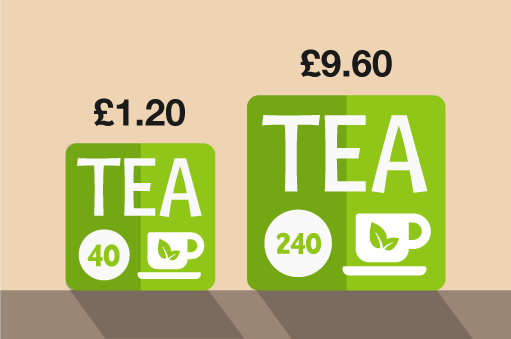Figure 20 Shopping options: tea

There are various ways of comparing the prices.

### Method 1

To work out which is the best value for money we need to find the price of 1 teabag.

If 40 teabags cost £1.20 then to find the cost of 1 teabag you do:

£1.20 ÷ 40 = £0.03, or 3p

If 240 teabags cost £9.60 then to find the cost of 1 teabag you do:

£9.60 ÷ 240 = £0.04, or 4p

The box containing 40 teabags is therefore better value than the larger box.

### Method 2

The ratio of teabags is

40 : 240 which you can simplify to 1:6

If we use the price for the small box you can see that 1 part is £1.20

You can then use this value to calculate the price of the large box. At this price, the bigger box would be £1.20 × 6 = £7.20 so we can see that the small box is better value.

## Activity 29: Practical applications of ratio

Use whichever method you prefer to work out the best deal in each case.

1. Work out which deal is the best value for money.

• a.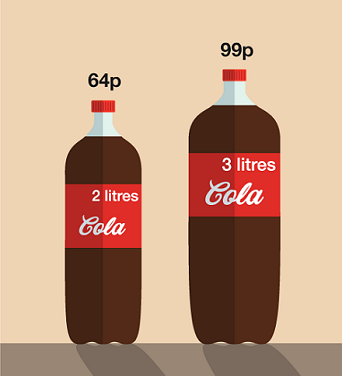Figure 21 Cola options

1.

• a.2 litres cost 64 p, so 1 litre costs 64 p ÷ 2 = 32p.

3 litres cost 99p, so 1 litre costs 99p ÷ 3 = 33p.

Comparing the cost of 1 litre in each case, we see that the 2-litre bottle is the best buy.

•

• b.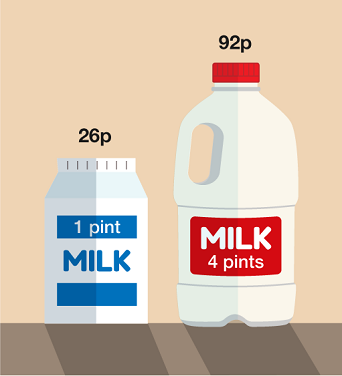Figure 22 Milk options

1.

• b.1 pint costs 26p.

4-pint carton costs 92p, so 1 pint costs 92p ÷ 4 = 23p.

Comparing the cost of 1 pint of milk in each case, we see that the 4-pint carton is the best buy.

•

• c.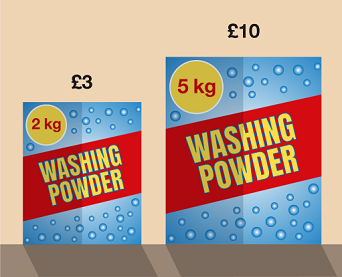Figure 23 Washing powder options

1.

• c.5 kg costs £10, so 1 kg costs £10 ÷ 5 = £2.

2 kg cost £3, so 1 kg costs £3 ÷ 2 = £1.50.

Comparing the cost of 1 kg of powder in each case, we see that the 2 kg box is the best buy.

1. Two supermarkets sell the same brand of juice. Shop B is offering ‘buy one get second one half price’ for apple juice and ‘buy one get one free’ for orange juice.

For each type of juice which shop is offering the best deal?

• a.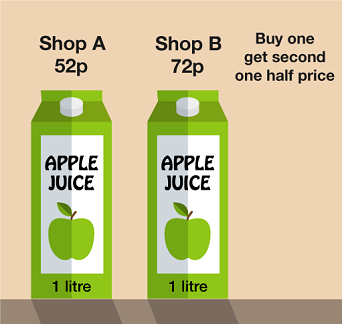Figure 24 Apple juice options

1.

• a.Shop A: 1 litre costs 52p

Shop B: 2 litres cost 72p + 36p = 108p (here we pay 72p for the first litre and 36p for second litre), so 1 litre costs 108p ÷ 2 = 54p.

Comparing the cost of 1 litre of apple juice in each case, we see that Shop A offers the better deal.

•

• b.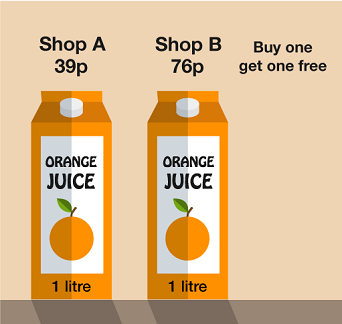Figure 25 Orange juice options

1.

• b.Shop A: 1 litre costs 39p

Shop B: 2 litres cost 76p (we get 1 litre free), so 1 litre costs: 76p ÷ 2 = 38p.

Comparing the cost of 1 litre of orange juice in each case, we see that Shop B offers the better deal.

1. A supermarket sells bread rolls in 3 different size packs. Which size offers the best value for money?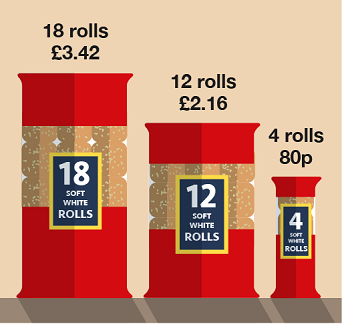1. Calculate the cost of 1 roll in each pack:

• 80p ÷ 4 = 20p

£2.16 ÷ 12 = £0.18 or 18p

£3.42 ÷ 18 = £0.19 or 19p

The pack of 12 is best value.

You have now completed all elements of the ratio section and hopefully are feeling confident with each topic.

The next section of the course deals with formulas. This might sound daunting but you have actually already used a formula. Remember when you learned about how to work out the percentage change of an item? To do that you used a simple formula and you will now take a closer look at slightly more complex formulas.

## Summary

In this section you have:

• learned about the three different types of ratio problems and that the aim of any ratio problem is to find out how much one part is worth

• practised solving each type of ratio problem:

• where the total amount is given
• where you are given the total of only one part
• where only the difference in amounts is given
• learned about other useful applications of ratio, such as changing the proportions of a recipe.

FSM_2_CYMRU#### Find out more

*Eligibility rules apply for financial support.